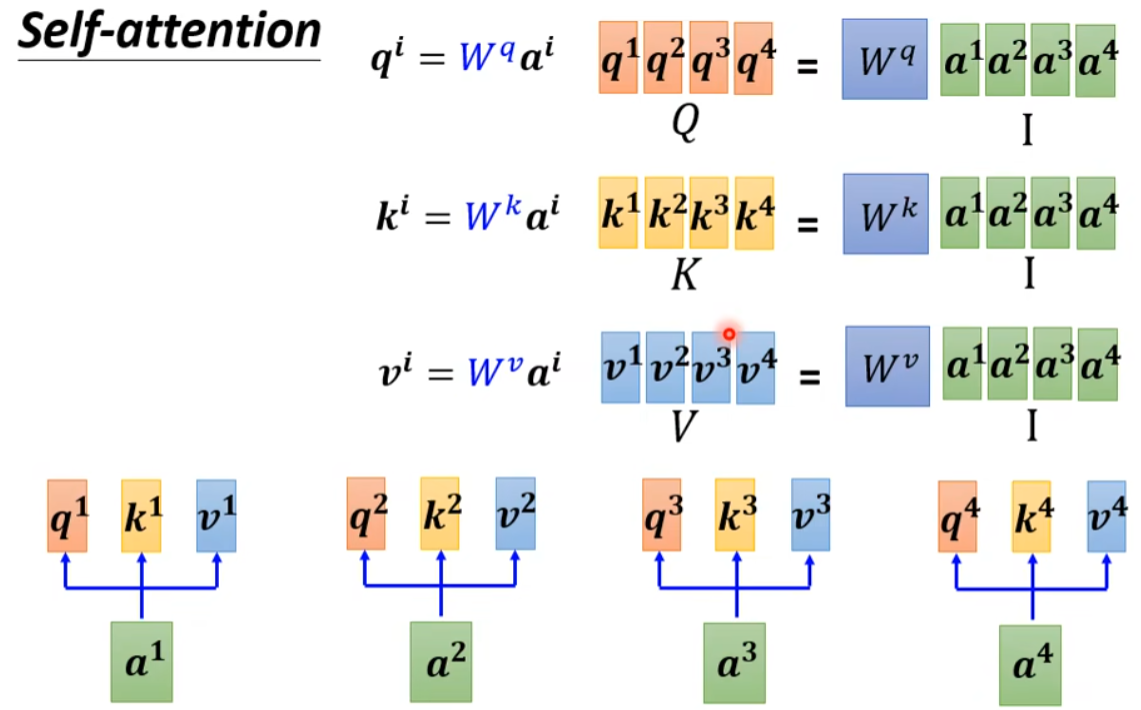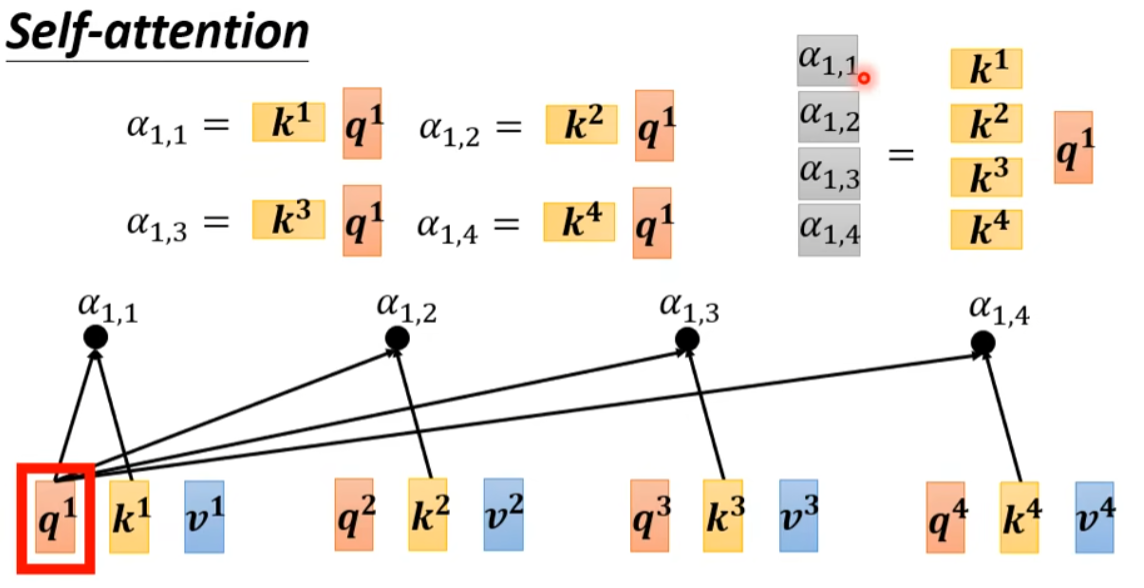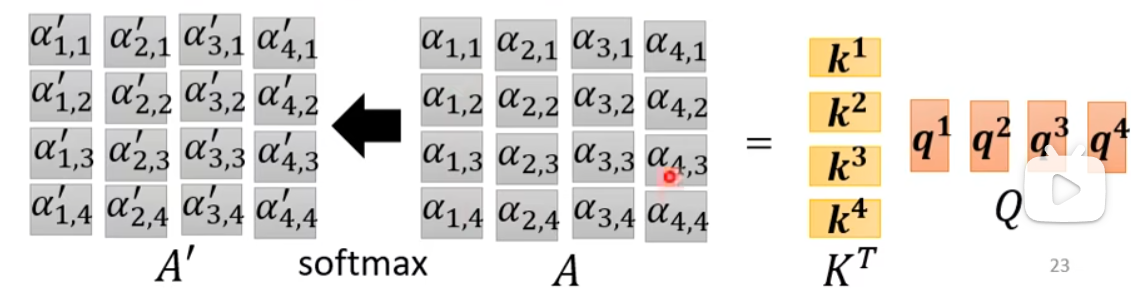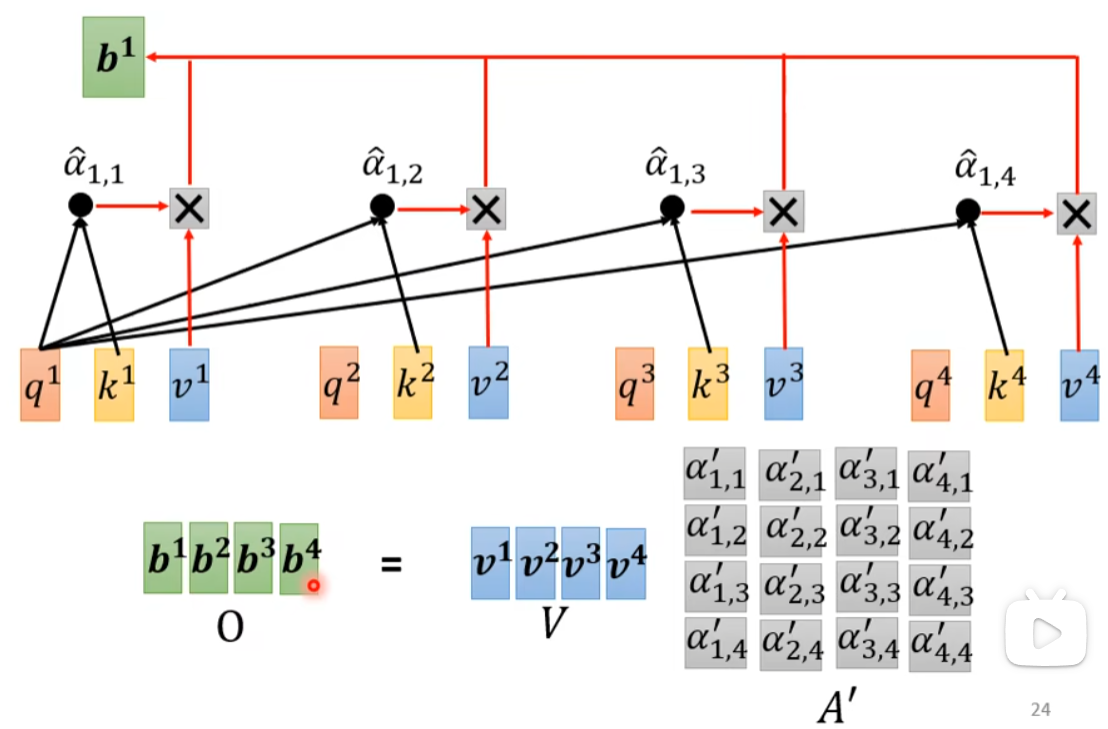0

# 【动手深度学习-笔记】注意力机制（四）自注意力、交叉注意力和位置编码

## 自注意力（Self-Attention）

     x

1

,

…

,

x

n

，

x

i

∈

R

d

\mathbf{x}_1, \ldots, \mathbf{x}_n，\mathbf{x}_i \in \mathbb{R}^d

x1​,…,xn​，xi​∈Rd，该序列的自注意力输出为一个长度相同的序列

y

1

,

…

,

y

n

\mathbf{y}_1, \ldots, \mathbf{y}_n

y1​,…,yn​，其中：

y

i

=

f

(

x

i

,

(

x

1

,

x

1

)

,

…

,

(

x

n

,

x

n

)

)

∈

R

d

\mathbf{y}_i = f(\mathbf{x}_i, (\mathbf{x}_1, \mathbf{x}_1), \ldots, (\mathbf{x}_n, \mathbf{x}_n)) \in \mathbb{R}^d

yi​=f(xi​,(x1​,x1​),…,(xn​,xn​))∈Rd

f

f

f是注意力汇聚函数，

f

f

f的第一个输入参数

x

i

\mathbf{x}_i

xi​作为query，剩下的参数为

x

\mathbf{x}

x自己和自己组成的键值对。


## 例子

    Q

、

K

、

V

\boldsymbol{Q}、\boldsymbol{K}、\boldsymbol{V}

Q、K、V的输入。这里的输入词元序列是

I

=

[

a

1

,

…

,

a

4

]

，

a

i

∈

R

d

\mathbf{I}=[\mathbf{a}_1, \ldots, \mathbf{a}_4]，\mathbf{a}_i \in \mathbb{R}^d

I=[a1​,…,a4​]，ai​∈Rd，

I

\mathbf{I}

I分别和三个矩阵

W

q

、

W

k

、

W

v

\boldsymbol{W}^{q}、\boldsymbol{W}^{k}、\boldsymbol{W}^{v}

Wq、Wk、Wv相乘得到

Q

、

K

、

V

\boldsymbol{Q}、\boldsymbol{K}、\boldsymbol{V}

Q、K、V，这里的

W

q

、

W

k

、

W

v

\boldsymbol{W}^{q}、\boldsymbol{W}^{k}、\boldsymbol{W}^{v}

Wq、Wk、Wv是可学习的参数。Q

\boldsymbol{Q}

Q中的每一个分量

q

i

\bold{q}_i

qi​，把它和

K

\boldsymbol{K}

K中所有的

k

i

\bold{k}_i

ki​相乘，得到注意力权重向量

α

i

\bold{\alpha}_i

αi​（图中的灰色部分）：q

i

\bold{q}_i

qi​得到的权重向量拼起来再通过softmax，得到的就是权重矩阵：V

\bold{V}

V相乘，得到最终的输出序列

O

\bold{O}

O，它的形状和输入序列

I

\bold{I}

I一致：W

q

、

W

k

、

W

v

\boldsymbol{W}^{q}、\boldsymbol{W}^{k}、\boldsymbol{W}^{v}

Wq、Wk、Wv是要学习的参数：## Self-Attention vs Convolution

On the Relationship between Self-Attention and Convolutional Layers

More generally, fully-attentional models seem to learn a generalization of CNNs where the kernel pattern is learned at the same time as the filters—similar to deformable convolutions.

## Self-Attention vs RNN

RNN的输入输出和自注意力很类似，都是输入一个序列输出一个序列。

RNN的特点在于，对于一个输入序列的某个词元，它只考虑该词元之前输入的词元信息（这里指最一般的单向RNN，双向RNN也是可以考虑双向信息的），且如果是一个很长的序列，那么开头的词元信息就很难保留到末尾的词元；

## 位置编码（Position Encoding，PE）

Transformer使用了三角位置编码：

         P

E

(

p

o

s

,

2

i

)

=

sin

⁡

(

p

o

s

/

1000

0

2

i

/

d

model

)

P

E

(

p

o

s

,

2

i

+

1

)

=

cos

⁡

(

p

o

s

/

1000

0

2

i

/

d

model

)

\begin{aligned} P E_{(p o s, 2 i)} &=\sin \left(p o s / 10000^{2 i / d_{\text {model }}}\right) \\ P E_{(p o s, 2 i+1)} &=\cos \left(p o s / 10000^{2 i / d_{\text {model }}}\right) \end{aligned}

PE(pos,2i)​PE(pos,2i+1)​​=sin(pos/100002i/dmodel ​)=cos(pos/100002i/dmodel ​)​


    p

o

s

pos

pos是词向量在序列中的位置，

i

i

i表示的是词向量内的第

i

i

i个分量，

d

m

o

d

e

l

d_{model}

dmodel​是词向量的长度。


    sin

⁡

\sin

sin编码，奇数维用

cos

⁡

\cos

cos编码，**这样编码的原因是基于三角函数的和差化积性质**：

sin

⁡

(

α

+

β

)

=

sin

⁡

α

cos

⁡

β

+

cos

⁡

α

sin

⁡

β

cos

⁡

(

α

+

β

)

=

cos

⁡

α

cos

⁡

β

−

sin

⁡

α

sin

⁡

β

\begin{aligned} \sin (\alpha+\beta)=\sin \alpha \cos \beta+\cos \alpha \sin \beta \\ \cos (\alpha+\beta)=\cos \alpha \cos \beta-\sin \alpha \sin \beta \end{aligned}

sin(α+β)=sinαcosβ+cosαsinβcos(α+β)=cosαcosβ−sinαsinβ​


    α

+

β

α+β

α+β的向量可以表示成位置

α

α

α和位置

β

β

β的向量组合，这提供了表达相对位置信息的可能性


    p

o

s

=

10

pos=10

pos=10可以和

p

o

s

=

1

,

p

o

s

=

9

pos=1, pos=9

pos=1,pos=9处的词向量建立联系，或者

p

o

s

=

2

,

p

o

s

=

8

pos=2,pos=8

pos=2,pos=8等。


## 视觉中的二维位置编码

DETR中，为了保留特征的空间信息，没有将二维数据平铺为一维，而是分别对行和列进行位置编码。

## 参考

10.6. 自注意力和位置编码 — 动手学深度学习 2.0.0-beta1 documentation

Jean-Baptiste Cordonnier et al. “On the Relationship between Self-Attention and Convolutional Layers…” Learning (2019): n. pag.

### “【动手深度学习-笔记】注意力机制（四）自注意力、交叉注意力和位置编码”的评论:

##### 关于作者##### overfit同步小助手